Mathematics For The International Student: Mathematics Sl - 3 Edition - Chapter 1 - Problem 15
Register Now

Join StudySoup

Get Full Access to Mathematics For The International Student: Mathematics Sl - 3 Edition - Chapter 1 - Problem 159781921972089

# A truck carrying a wide load needs to pass through the parabolic tunnel shown. The units

Mathematics for the International Student: Mathematics SL | 3rd Edition

Problem 15

A truck carrying a wide load needs to pass through the parabolic tunnel shown. The units are metres. The truck is $$5 \mathrm{~m}$$ high and $$4 \mathrm{~m}$$ wide. a) Find the quadratic function which describes the shape of the tunnel. b) Determine whether the truck will fit.

Accepted Solution
Step-by-Step Solution:

Step 1 of  2

a)  Consider the figure above,

The parabola cuts x-axis at -3 and 3.

We have conjugate (x-3) and (x+3)

Then ,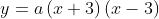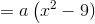Parabola passes through (0,8)

So,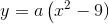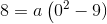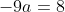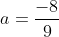The quadratic function which describes the shape of tunnel is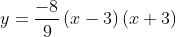###### Chapter 1, Problem 15 is Solved

Step 2 of 2

Unlock Textbook Solution